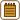## Heating the Log Cabin

### Task number: 1797

a) How much heat transfers through side walls of a log cabin during one winter day? Length of the cabin is 10 m, width is 7 m, height of the walls is 3.5 m and their thickness is 50 cm. Average outdoor temperature is -10 °C and the indoor temperature is kept at 18 °C.

b) How much wood has to be burnt up during one day in a stove, whose thermal efficiency is 30%, so that the indoor temperature is being kept constant?

c) How much would an electric heating of the cabin cost? Efficiency of electric heating is practically 100% and an average price of electric energy is for example 4.30 CZK/kWh.

d) How high must the volumetric flow rate of water in the radiator be if water temperature at the entry into the radiator is 80 °C and temperature of the water leaving the radiator is 70 °C?

Assume that the roof is so well thermally isolated that we can neglect heat loss through the roof.

• #### Hint

Transferred heat can be calculated from Fourier’s law of heat conduction.

• #### Notation

 a = 10 m length of the cabin b = 7 m width of the cabin h = 3.5 m height of the cabin walls d = 50 cm  = 0.50 m thickness of the cabin walls t1 = -10 oC average outdoor temperature t2 = 18 oC indoor temperature t3 = 80 oC water temperature at the entry into the radiator t4 = 70 oC temperature of the water leaving the radiator τ = 1 d  = 86 400 s time η = 30% = 0.3 wood stove efficiency Q = ? heat transferred through the walls m = ? mass of wood to be burnt qV = ? volumetric flow rate of water in the radiator From the Handbook of Chemistry and Physics: λ = 0.15 W m-1K-1 thermal conductivity of wood H = 15 MJ kg-1 heat of combustion for wood cwater = 4 180 J kg-1 K-1 specific heat capacity of water
• #### Analysis

The heat transferred through a homogeneous board (in our case, the wall; inhomogeneity of the wall such as windows and the door are not considered) is proportional to the area of the wall (board), time, during which the heat is being transferred, and the temperature difference between the ends of the board (under the condition that the temperature difference is constant). On the contrary, the transferred heat is inversely proportional to the thickness of the board (wall). For some materials their ability to transfer the heat is specified by so-called thermal conductivity. The greater it is the more heat is being transferred. To calculate the heat transferred through the walls during one day, we have all the required values.

To keep the indoor temperature constant, we have to supply the same heat that is being transferred to the environment. To calculate the required amount of wood, we will use its heat of combustion, i.e. the heat we receive by burning 1 kg of wood.

Using the fact that the water has to supply so much heat so that the indoor temperature is kept constant, we calculate the required volumetric flow rate of water in the radiator. Supplied heat is proportional to the difference between the temperature of flowing in water and the water leaving the radiator.

• #### Solution

a) For transferring heat it holds that:

$Q=\lambda \frac{S \tau}{d} \Delta t\,,$

where λ is the thermal conductivity of wood, S is the total area of the walls, for which it is true that

$S=(2a+2b)h\,,$

where d is the thickness of the walls, τ is the period of time during which the heat has been transferring and Δt = t2t1 is the difference between the temperature inside and outside the cabin.

By substitution we obtain the relation, which we can substitute given values into:

$Q=\lambda\frac{\left(2a+2b\right)h\tau}{d}\left(t_2-t_1\right)\,,$ $Q=0.15\cdot\frac{\left(2\cdot{10}+2\cdot{7}\right)\cdot3.5\cdot{86400}}{0.5}\cdot\left[18-(-10)t_1\right]\,\mathrm{J}\,,$ $Q\dot{=}8.6\cdot{10}^{7}\,\mathrm{J}=86\,\mathrm{MJ}\,.$

b) Burning the wood whose mass is m, we obtain the heat Hm. However, only η = 30% of this heat we use for heating the cabin (wood stove efficiency). The heat used for heating the cabin has to equal the heat transferred through the walls, therefore

$Q=\eta Hm\,,$

Hence we will express the required mass of wood m and calculate it by substitution:

$m=\frac{Q}{\eta H}=\frac{86\,\mathrm{MJ}}{0.3\cdot{15}\,\mathrm{MJ\cdot kg^{-1}}}\dot{=}19\,\mathrm{kg}\,.$

c) First we derive the conversion relationship between the units of energy:

$1\,\mathrm{kWh}=1\,\mathrm{kW}\cdot1\,\mathrm{h}=1000\,\mathrm{W}\cdot\,3600\,\mathrm{s}=3.6\cdot{10^6}\,\mathrm{Ws}=3.6\,\mathrm{MJ}\,.$

From the conversion relationship we see that 3.6 MJ of electric energy cost 4.30 CZK. It means that the amount of money we would have paid for the electric heating for one day is:

$\frac{86}{3.6}\cdot4.30\,\mathrm{CZK}=103\,\mathrm{CZK}\,.$

d) If we denote the volumetric flow rate of water in the radiator as qV, then the volume of the water passed through the radiator during the time τ is qVτ. This water cools down and supplies the heat

$Q_V=cq_V\tau \left(t_3-t_4\right)\,.$

Then we compare the heat supplied by water and the heat transferred through the walls

$Q_V=Q\,,$ $c_{\mathrm{water}}q_V\tau\left(t_3-t_4\right)=\lambda\frac{\left(2a+2b\right)h\tau}{d}\left(t_2-t_1\right)\,.$

and express the unknown volumetric flow rate and substitute given values:

$q_V=\lambda\frac{\left(2a+2b\right)h\left(t_2-t_1\right)}{c_{\mathrm{water}}d\left(t_3-t_4\right)}\,,$ $q_V=0.15\cdot\frac{\left(2\cdot{10}+2\cdot{7}\right)\cdot{3.5}\cdot\left[18-(-10)\right]}{4180\cdot{0.5}\cdot\left(80-70\right)}\,\mathrm{kg\cdot s^{-1}}\,,$ $q_V\dot{=}0.024\,\mathrm{kg\cdot s^{-1}}=86\,\mathrm{kg\cdot h^{-1}}\,.$